# Filtering a List into Unique Records Using the COUNTIF Formula in Microsoft Excel

If you have a range of values which contain duplicates, we have an interesting way of filtering for these duplicate values. This is one of the ways of obtaining a list of duplicates and then either highlighting or deleting them as the need may be.

How to use excel functions to find the duplicates in data?

So first we will identify the duplicate rows. There are many ways of doing this and this is just one of the methods to identify using formula.

The formula we need to use is –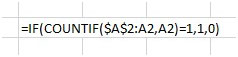You will observe that in the formula =IF(COUNTIF(\$A\$2:A2,A2)=1,1,0),

In the highlighted section the first instance of A2 is shown as \$A\$2 and the other is just A2. Well, we want to count from the beginning of the range which is A2 going down one row each time. So that’s why the 1st instance is absolute and the next is relative. This way, when you drag the formula down, from B2 to B3, the formula will change to =IF(COUNTIF(\$A\$2:A3,A3)=1,1,0) and so on.

The 2nd parameter A2 (highlighted in green) is the criteria for the countif function.

So we compare if the count = 1, if yes, show 1 in column B for that row, else show 0. So if the count is more than 1 as in the case of duplicates, it will show 0 here.

This is our data set before applying the formula –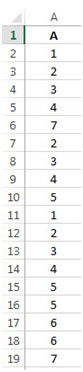So after applying this formula in the entire column, we get the following results –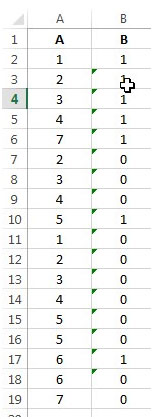Now we find that all the unique values are marked as 1 and the duplicates are marked as 0 in column B. So if we need the unique values, we need to apply the filter to the columns by clicking on the Data menu and click on Filter in the Sort & Filter group.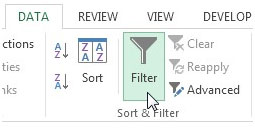The filter has been applied –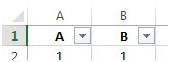Then filter by 1 in column B and highlight it or copy it to another sheet as per the requirement.

If we want the duplicate values to be highlighted or deleted, we need to filter by 0 –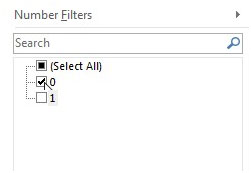You can see all the values being filtered. Now you can either delete these rows as they are duplicates or color them, depending on your need.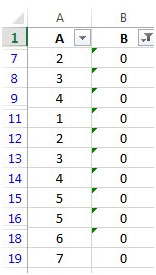So this is how a formula helps us in identifying duplicates and then taking the required action on them.

1.Based on what is selected in column A from a dropdown, I want Column B, to give them another specific group of choices from a separate dropdown? Any help is appreciated

2."Alan,

You are a life saver (no, not the candy).

That did it (with a little tweaking for exactly what I wanted)!

Thanks.

Ryan"

3."Hi Ryan,

You should still be able to use that same basic formula, but with a slight tweak.

If columns B and C contain the data you gave, and A1 contains, the name of the athlete, then the following should do it:

{=SUM(((B1:B6)=A1)*((C1:C6)=""W""))}

A3 might then have:

{=SUM(((B1:B6)=A1)*((C1:C6)=""L""))}

If you want to display the text as you outlined above you can do that by either concatenation or using a custom format.

Does that do it?

Alan."

4."On the right track, but it's not right yet.

Let me be more specific to make it easier. There will be multiple entries for any single athlete, but the result will obviously change depending on success.
Names are in column B and Results are in Column C
John Smith W
Jack Johnson L
Pete Anderson W
John Smith L
John Smith W
Pete Anderson L

I want to add up the records in Cell A2 (with a CONCATENATE command) by a dropdown list of athletes names that I've created with everyone's name (spelled correctly) in Cell A1.

Cell A1 will have a dropdown of
John Smith
Jack Johnson
Pete Anderson
etc...

Cell A2 will have
Wins 2 (or whatever)

Cell A3 will have
Losses 2 (copy of A2 referencing losses)

I really appreciate all of your help Alan. If you would also email me directly at ryan_molitor@hotmail.com, that would also help considerably..."

5."Hi Rmolitor,

COUNTIF is really just a fudge functon that tries to make it easy for people to build an array formula withouth knowing anything about what an array formula is.

As soon as you get into a situation where you need to use multiple criteria, you only have two options that I can see:

1) Create some ugly new field that people often call something like a ""unique identifier"" - normally by concatenating other fields.

This is both ugly and unecessary.

2) Use and array formula to multiply (effectively create AND operators) across multiple fields:

Example Data:

Alan Red 1
Alan Blue 2
Bob Blue 3
Bob Red 4

The following formula returns the value '2':

{=SUM((A1:A4=""Alan"")*(B1:B4=""Blue"")*(C1:C4))}

This has to be entered, without the braces, using Shift-Ctrl-Enter.

Alan."

6."I have an athletic spreadsheet displaying the name and result of a particular match. I want to be able to Auto Filter the names to easily read the results. That's easy...

Now I want to also be able to count the ""Wins"" and ""Losses"" of that particular athlete without adding up all of the others stats.

The Auto Filter just simply ""hides"" the others names when I choose a particular athlete so when I do a COUNTIF, it counts every name rather than the filtered name. Is there a secret COUNTIF IF statement that I can embed?"

7."Hi Jorge,

You don't say how you would like to be warned, so I will assumed that Column B is empted, and we will make the cell beside your new entry in Column C change to ""Repeat!"" if it is so:

Assuming your list starts in C1 and goes down, enter the following in B1, and copy down as you enter new names:

=IF(COUNTIF(C\$1:C1,C1)=1,"""",""Repeat!"")

Notes:

If you copy it past the end of your list, into a blank part of the sheet, it will flag repeats of the blank cells, so you might want to error trap if you need to go that way.

This will only work if you enter the names with exactly the same spelling and form of course.

Alan."

8.I am trying to create a spreadsheet which contains names of people on column C. However, as I enter names into the cells in column C, I would like excel to give me an error if the name being entered already exists in another cell in Column C. Anyone know how to do that?

9."Note that if you use less than or equal to for the later date boundry, then it will include that date (depends on what you want exactly).

The issue is with the signs. The board interprets them as HTML code. I think the IRUBIN conslutants are looking at it.

Alan."

10."This interface keeps reversing the ""less than or equal to"" (=VALUE(""1 Aug 2003""))*((A2:A45)<=VALUE(""15 Aug 2003"")))} "

11."Hi Alan--

Brilliant! With a few minor adjustments, the following works correctly:

{=SUM(((A2:A45)>=VALUE(""1 Aug 2003""))*((A2:A45)<=VALUE(""16 Aug 2003"")))}

Thank you thank you thank you!"

12."Hi Scott,

I tested it again and it definately works.

Are you sure you are entering the full formula - not just what appears above (note my repeated comments regarding the missing elements that the ExcelTip board refuses to post)?

I'll try again here, but I am not hopeful:

{=SUM(((A1:A4)>=VALUE(""1 Aug 2003""))*
((A1:A4)
If that appears correctly, it should contain 69 characters from the opening curly bracket to the closing one inclusive.

If you have entered the formula *fully*, and it still doesn't work, you might want to read the Excel help file on array formulae to see if you are entering it correctly.

If that doesn't appear correctly, I am giving up and someone else can help Scott with the 'correct' answer.

My offer is withdrawn - please do not PM me.

Alan."

13."Hi Alan--

Does anybody else have the correct answer?"

14."Hi Scott,

I think that formula up above would solve your problems, but the board consistently cuts off the formula for some reason.

Same offer is still open as per my reply (August 15, 2003 9:58 PM EST).

Alan."

15."Hi Alan--

Thanks for all your help thus far. I'm sure you have other things to do besides answering my silly little questions. As I stated in my original post from Aug 15, ""I need to count the number of times that the dates from Aug 1, 2003 THROUGH Aug 15, 2003 occur in a column."" To answer your question above, no, I'm not counting ""y""s. I need to count the NUMBER of dates from 08/01/03 to and including 08/15/03. COUNTIF works beautifully if I only want to start counting from 1 Aug 2003 and include every date on up. For instance, if I use =COUNTIF(A2:A182,"">=1 AUG 2003"") I get 181 returned. However, the delimiting part <=15 AUG 2003 is still a mystery. Where does that go in the equation? Do you know? I've tried various versions of nested functions, but I keep getting the ""Too many arguments"" error message. Ugh!"

16."Hi Scott,

Why can't that be right?

If you hve only two 'y's and tey had, say, 6850000 and 537 beside them, then you'd get that answer (or example).

Do you mean you want to count the NUMBER of 'y's? If so, just use COUNTIF.

Alan."

17."Oooops! I noticed an error in the formula and thought I had it fixed by using {=SUM(((A2:A182)>=VALUE(""1 Aug 2003""))*((A2:A182)))}
It returned 6850537! This, of course, is incorrect. Even if every date in that column were between 1 Aug through 15 Aug, it cannot be any higher than 181, since I'm only using 181 cells. (Manually counting, the correct answer should be 101.) Help!"

18."Sorry Alan

Your solution, corrected by Excel, gives me a #VALUE! error. I used {=SUM(((A2:A182)>=VALUE(""1 Aug 2003""))*((A1:A4)))} Is there more to the formula? I noticed you used >=VALUE in your formula. I'm trying to delimit the date range from 1 Aug 2003 through 15 Aug 2003. Shouldn't <=VALUE be included somewhere as well? Also, I'm using the 08/01/03 date format. Does that make any difference?"

19."Hi Casey,

If that data is in A1:B4, then:

{=SUM((A1:A4=""y"")*(B1:B4))} equals 60
{=SUM((A1:A4=""n"")*(B1:B4))} equals 80

Note that these are array formulae, and must be entered (without the curly brackets), with Shift-Ctrl-Enter.

Check the Excel help for more on arrays.

Alan."

20."i am trying to sum certain numbers in one column based on data from another column. for instance:
column1 column2
y 20
n 30
y 40
n 50

i want to be able to sum the y's and sum the n's..thanks"

21."Not sure what is going wrong - I cannot seem to get the whole formula in.

Ah well, try again:

{=SUM(((A1:A4)>=VALUE(""1 Aug 2003""))*((A1:A4)
If that looks the same as above, then the following should end the formula:

If that doesn't work, then PM me from the forums section.

Alan."

22."Looks like the forumula got cut off when I pasted it. Should read:

{=SUM(((A1:A4)>=VALUE(""1 Aug 2003""))*((A1:A4)
Sorry!

Alan."

23."Hi Scott,

Lets assumed you have A1:A4 containing:

7 Jul 2003
3 Aug 2003
13 Aug 2003
16 Aug 2003

The following formula will return the value '2':

{=SUM(((A1:A4)>=VALUE(""1 Aug 2003""))*((A1:A4)
Note that this is an array formula, so you must enter it (without the curly brackets), with Shift-Ctrl-Enter.

Check Excel's help for more on array formulae.

Does that work?

Alan."

24.I need to count the number of times that the dates from Aug 1, 2003 THROUGH Aug 15, 2003 occur in a column. Is this possible?

25."Hi Sean,

I don't really understand what you mean.

The formulae immediately above in my reply to 'jonny' will give you a way to identify unique entries (count of 1), and if you dropped that '1' into column B, and then summed column B it would give a count of total unique entries.

However, I don't understand what you mean by, ""based on other field in the adjcent rows"".

Please can you elaborate or give a short example of the data and result you want?

Thanks,

Alan."

26."Hi jonny,

If A1:A99 contains a list of items, some of which are duplicates, then enter the following formula in B1 and copy down to the end of the list:

=COUNTIF(A\$1:\$A99,A1)

This will return a value that tells you how many times the entry in column A appears in the list.

If you want to go further and actually display that duplicate enter beside itself, but show nothing for entries that only occur once, you could expand the formula to:

=IF(COUNTIF(A\$1:A\$4,A1)=1,"""",A1)

That will show only the entries that appears more than once.

If you want to expand the range from a single column (A) to a list / block of items across, say, three columns (A1:C99), then in column D, enter:

=IF(COUNTIF(\$A\$1:\$C\$99,A1)=1,"""",A1)

Copy this across to E and F, and down to the bottom of the list, and the formulae will give you items that appear in the block of data more than once.

Not sure if that is what you mean, but hopefully one or more of the above will allow you to extrapolate to get what you actually need.

Alan."

27.I am trying to count the number of unique entries in a column based on other field in the adjcent rows

28.I NEED TO FIND THE DUPLICATE VALUES IN 3 COLUMNS AND JUST DISPLAY DUPICATE IN ANOTHER COLUMN. HOW DO I DO DO THAT

29.Just use COUNTIF then set the filter to 1

30.Use the Advanced Filter and click on 'Unique Records Only'. Much quicker!!

31.this formula with iF AND COUNTIF is excelent. The formulas: =A1=A2 in the same table, select FALSE in the criteria list of filter. =if(A1A2,0,1) is functional too select 0 in the criteria list NOTE. ordered data

Terms and Conditions of use

The applications/code on this site are distributed as is and without warranties or liability. In no event shall the owner of the copyrights, or the authors of the applications/code be liable for any loss of profit, any problems or any damage resulting from the use or evaluation of the applications/code.